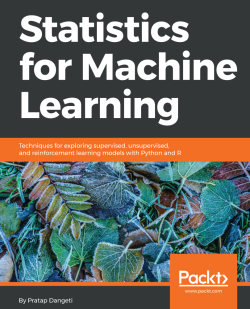# Statistics for Machine Learning4.2 (20 reviews total)
By Pratap Dangeti
• \$41.99 eBook
• \$12.99 eBook + Subscription
What do you get with a Packt Subscription?

• Constantly updated with 100+ new titles each month
• Breadth and depth in over 1,000+ technologies

Complex statistics in Machine Learning worry a lot of developers. Knowing statistics helps you build strong Machine Learning models that are optimized for a given problem statement. This book will teach you all it takes to perform complex statistical computations required for Machine Learning. You will gain information on statistics behind supervised learning, unsupervised learning, reinforcement learning, and more. Understand the real-world examples that discuss the statistical side of Machine Learning and familiarize yourself with it. You will also design programs for performing tasks such as model, parameter fitting, regression, classification, density collection, and more.

By the end of the book, you will have mastered the required statistics for Machine Learning and will be able to apply your new skills to any sort of industry problem.

Publication date:
July 2017
Publisher
Packt
Pages
442
ISBN
9781788295758

## Chapter 1. Journey from Statistics to Machine Learning

In recent times, machine learning (ML) and data science have gained popularity like never before. This field is expected to grow exponentially in the coming years. First of all, what is machine learning? And why does someone need to take pains to understand the principles? Well, we have the answers for you. One simple example could be book recommendations in e-commerce websites when someone went to search for a particular book or any other product recommendations which were bought together to provide an idea to users which they might like. Sounds magic, right? In fact, utilizing machine learning, can achieve much more than this.

Machine learning is a branch of study in which a model can learn automatically from the experiences based on data without exclusively being modeled like in statistical models. Over a period and with more data, model predictions will become better.

In this first chapter, we will introduce the basic concepts which are necessary to understand both the statistical and machine learning terminology necessary to create a foundation for understanding the similarity between both the streams, who are either full-time statisticians or software engineers who do the implementation of machine learning but would like to understand the statistical workings behind the ML methods. We will quickly cover the fundamentals necessary for understanding the building blocks of models.

In this chapter, we will cover the following:

• Statistical terminology for model building and validation
• Machine learning terminology for model building and validation
• Machine learning model overview

## Statistical terminology for model building and validation

Statistics is the branch of mathematics dealing with the collection, analysis, interpretation, presentation, and organization of numerical data.

Statistics are mainly classified into two subbranches:

• Descriptive statistics: These are used to summarize data, such as the mean, standard deviation for continuous data types (such as age), whereas frequency and percentage are useful for categorical data (such as gender).
• Inferential statistics: Many times, a collection of the entire data (also known as population in statistical methodology) is impossible, hence a subset of the data points is collected, also called a sample, and conclusions about the entire population will be drawn, which is known as inferential statistics. Inferences are drawn using hypothesis testing, the estimation of numerical characteristics, the correlation of relationships within data, and so on.

Statistical modeling is applying statistics on data to find underlying hidden relationships by analyzing the significance of the variables.

### Machine learning

Machine learning is the branch of computer science that utilizes past experience to learn from and use its knowledge to make future decisions. Machine learning is at the intersection of computer science, engineering, and statistics. The goal of machine learning is to generalize a detectable pattern or to create an unknown rule from given examples. An overview of machine learning landscape is as follows: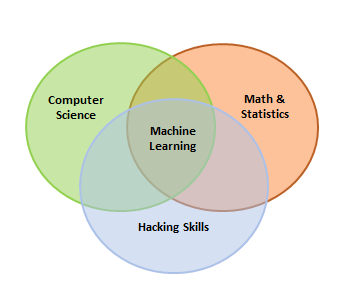Machine learning is broadly classified into three categories but nonetheless, based on the situation, these categories can be combined to achieve the desired results for particular applications:

• Supervised learning: This is teaching machines to learn the relationship between other variables and a target variable, similar to the way in which a teacher provides feedback to students on their performance. The major segments within supervised learning are as follows:
• Classification problem
• Regression problem
• Unsupervised learning: In unsupervised learning, algorithms learn by themselves without any supervision or without any target variable provided. It is a question of finding hidden patterns and relations in the given data. The categories in unsupervised learning are as follows:
• Dimensionality reduction
• Clustering
• Reinforcement learning: This allows the machine or agent to learn its behavior based on feedback from the environment. In reinforcement learning, the agent takes a series of decisive actions without supervision and, in the end, a reward will be given, either +1 or -1. Based on the final payoff/reward, the agent reevaluates its paths. Reinforcement learning problems are closer to the artificial intelligence methodology rather than frequently used machine learning algorithms.

In some cases, we initially perform unsupervised learning to reduce the dimensions followed by supervised learning when the number of variables is very high. Similarly, in some artificial intelligence applications, supervised learning combined with reinforcement learning could be utilized for solving a problem; an example is self-driving cars in which, initially, images are converted to some numeric format using supervised learning and combined with driving actions (left, forward, right, and backward).

### Major differences between statistical modeling and machine learning

Though there are inherent similarities between statistical modeling and machine learning methodologies, sometimes it is not obviously apparent for many practitioners. In the following table, we explain the differences succinctly to show the ways in which both streams are similar and the differences between them:

 Statistical modeling Machine learning Formalization of relationships between variables in the form of mathematical equations. Algorithm that can learn from the data without relying on rule-based programming. Required to assume shape of the model curve prior to perform model fitting on the data (for example, linear, polynomial, and so on). Does not need to assume underlying shape, as machine learning algorithms can learn complex patterns automatically based on the provided data. Statistical model predicts the output with accuracy of 85 percent and having 90 percent confidence about it. Machine learning just predicts the output with accuracy of 85 percent. In statistical modeling, various diagnostics of parameters are performed, like p-value, and so on. Machine learning models do not perform any statistical diagnostic significance tests. Data will be split into 70 percent - 30 percent to create training and testing data. Model developed on training data and tested on testing data. Data will be split into 50 percent - 25 percent - 25 percent to create training, validation, and testing data. Models developed on training and hyperparameters are tuned on validation data and finally get evaluated against test data. Statistical models can be developed on a single dataset called training data, as diagnostics are performed at both overall accuracy and individual variable level. Due to lack of diagnostics on variables, machine learning algorithms need to be trained on two datasets, called training and validation data, to ensure two-point validation. Statistical modeling is mostly used for research purposes. Machine learning is very apt for implementation in a production environment. From the school of statistics and mathematics. From the school of computer science.

### Steps in machine learning model development and deployment

The development and deployment of machine learning models involves a series of steps that are almost similar to the statistical modeling process, in order to develop, validate, and implement machine learning models. The steps are as follows:

1. Collection of data: Data for machine learning is collected directly from structured source data, web scrapping, API, chat interaction, and so on, as machine learning can work on both structured and unstructured data (voice, image, and text).
2. Data preparation and missing/outlier treatment: Data is to be formatted as per the chosen machine learning algorithm; also, missing value treatment needs to be performed by replacing missing and outlier values with the mean/median, and so on.
3. Data analysis and feature engineering: Data needs to be analyzed in order to find any hidden patterns and relations between variables, and so on. Correct feature engineering with appropriate business knowledge will solve 70 percent of the problems. Also, in practice, 70 percent of the data scientist's time is spent on feature engineering tasks.
4. Train algorithm on training and validation data: Post feature engineering, data will be divided into three chunks (train, validation, and test data) rather than two (train and test) in statistical modeling. Machine learning are applied on training data and the hyperparameters of the model are tuned based on validation data to avoid overfitting.

1. Test the algorithm on test data: Once the model has shown a good enough performance on train and validation data, its performance will be checked against unseen test data. If the performance is still good enough, we can proceed to the next and final step.
2. Deploy the algorithm: Trained machine learning algorithms will be deployed on live streaming data to classify the outcomes. One example could be recommender systems implemented by e-commerce websites.

### Statistical fundamentals and terminology for model building and validation

Statistics itself is a vast subject on which a complete book could be written; however, here the attempt is to focus on key concepts that are very much necessary with respect to the machine learning perspective. In this section, a few fundamentals are covered and the remaining concepts will be covered in later chapters wherever it is necessary to understand the statistical equivalents of machine learning.

Predictive analytics depends on one major assumption: that history repeats itself!

By fitting a predictive model on historical data after validating key measures, the same model will be utilized for predicting future events based on the same explanatory variables that were significant on past data.

The first movers of statistical model implementers were the banking and pharmaceutical industries; over a period, analytics expanded to other industries as well.

Statistical models are a class of mathematical models that are usually specified by mathematical equations that relate one or more variables to approximate reality. Assumptions embodied by statistical models describe a set of probability distributions, which distinguishes it from non-statistical, mathematical, or machine learning models

Statistical models always start with some underlying assumptions for which all the variables should hold, then the performance provided by the model is statistically significant. Hence, knowing the various bits and pieces involved in all building blocks provides a strong foundation for being a successful statistician.

In the following section, we have described various fundamentals with relevant codes:

• Population: This is the totality, the complete list of observations, or all the data points about the subject under study.
• Sample: A sample is a subset of a population, usually a small portion of the population that is being analyzed.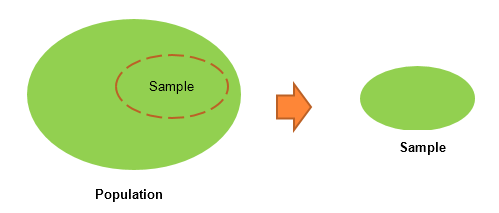### Note

Usually, it is expensive to perform an analysis on an entire population; hence, most statistical methods are about drawing conclusions about a population by analyzing a sample.

• Parameter versus statistic: Any measure that is calculated on the population is a parameter, whereas on a sample it is called a statistic.
• Mean: This is a simple arithmetic average, which is computed by taking the aggregated sum of values divided by a count of those values. The mean is sensitive to outliers in the data. An outlier is the value of a set or column that is highly deviant from the many other values in the same data; it usually has very high or low values.
• Median: This is the midpoint of the data, and is calculated by either arranging it in ascending or descending order. If there are N observations.
• Mode: This is the most repetitive data point in the data: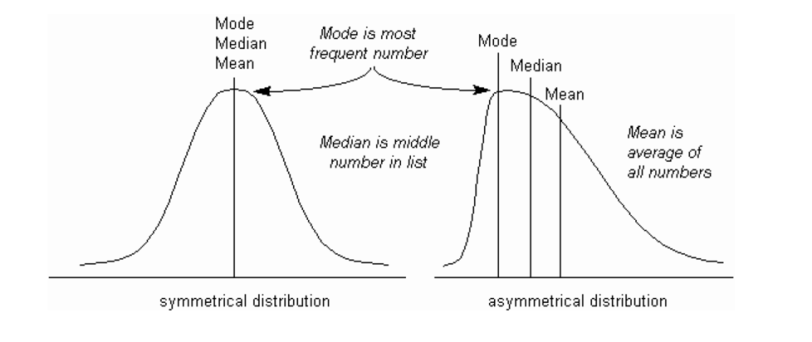The Python code for the calculation of mean, median, and mode using a `numpy` array and the `stats` package is as follows:

```>>> import numpy as np
>>> from scipy import stats

>>> data = np.array([4,5,1,2,7,2,6,9,3])

# Calculate Mean
>>> dt_mean = np.mean(data) ; print ("Mean :",round(dt_mean,2))

# Calculate Median
>>> dt_median = np.median(data) ; print ("Median :",dt_median)

# Calculate Mode
>>> dt_mode =  stats.mode(data); print ("Mode :",dt_mode)   ```

The output of the preceding code is as follows: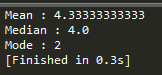### Note

We have used a NumPy array instead of a basic list as the data structure; the reason behind using this is the `scikit-learn` package built on top of NumPy array in which all statistical models and machine learning algorithms have been built on NumPy array itself. The `mode` function is not implemented in the `numpy` package, hence we have used SciPy's `stats` package. SciPy is also built on top of NumPy arrays.

The R code for descriptive statistics (mean, median, and mode) is given as follows:

```data <- c(4,5,1,2,7,2,6,9,3)
dt_mean = mean(data) ; print(round(dt_mean,2))
dt_median = median (data); print (dt_median)

func_mode <- function (input_dt) {
unq <- unique(input_dt)  unq[which.max(tabulate(match(input_dt,unq)))]
}

dt_mode = func_mode (data); print (dt_mode)```

### Note

We have used the default `stats` package for R; however, the `mode` function was not built-in, hence we have written custom code for calculating the mode.

• Measure of variation: Dispersion is the variation in the data, and measures the inconsistencies in the value of variables in the data. Dispersion actually provides an idea about the spread rather than central values.
• Range: This is the difference between the maximum and minimum of the value.
• Variance: This is the mean of squared deviations from the mean (xi = data points, μ = mean of the data, N = number of data points). The dimension of variance is the square of the actual values. The reason to use denominator N-1 for a sample instead of N in the population is due the degree of freedom. 1 degree of freedom lost in a sample by the time of calculating variance is due to extraction of substitution of sample: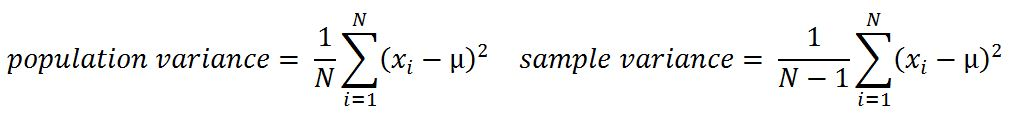• Standard deviation: This is the square root of variance. By applying the square root on variance, we measure the dispersion with respect to the original variable rather than square of the dimension: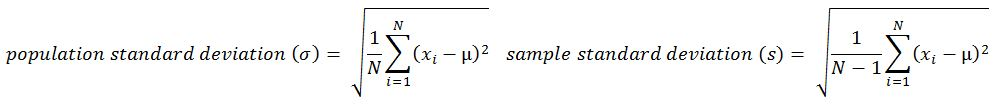• Quantiles: These are simply identical fragments of the data. Quantiles cover percentiles, deciles, quartiles, and so on. These measures are calculated after arranging the data in ascending order:
• Percentile: This is nothing but the percentage of data points below the value of the original whole data. The median is the 50th percentile, as the number of data points below the median is about 50 percent of the data.
• Decile: This is 10th percentile, which means the number of data points below the decile is 10 percent of the whole data.
• Quartile: This is one-fourth of the data, and also is the 25th percentile. The first quartile is 25 percent of the data, the second quartile is 50 percent of the data, the third quartile is 75 percent of the data. The second quartile is also known as the median or 50th percentile or 5th decile.
• Interquartile range: This is the difference between the third quartile and first quartile. It is effective in identifying outliers in data. The interquartile range describes the middle 50 percent of the data points.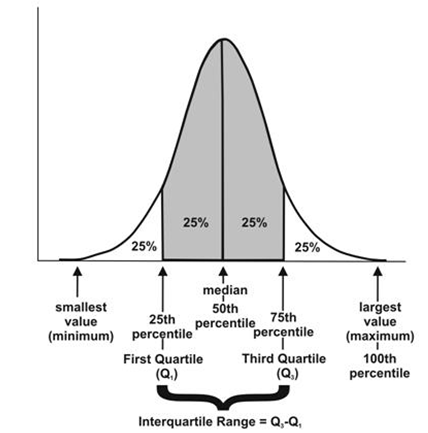The Python code is as follows:

```>>> from statistics import variance, stdev
>>> game_points = np.array([35,56,43,59,63,79,35,41,64,43,93,60,77,24,82])

# Calculate Variance
>>> dt_var = variance(game_points) ; print ("Sample variance:", round(dt_var,2))

# Calculate Standard Deviation
>>> dt_std = stdev(game_points) ; print ("Sample std.dev:", round(dt_std,2))

# Calculate Range
>>> dt_rng = np.max(game_points,axis=0) - np.min(game_points,axis=0) ; print ("Range:",dt_rng)

#Calculate percentiles
>>> print ("Quantiles:")
>>> for val in [20,80,100]:
>>>      dt_qntls = np.percentile(game_points,val)
>>>      print (str(val)+"%" ,dt_qntls)

# Calculate IQR
>>> q75, q25 = np.percentile(game_points, [75 ,25]); print ("Inter quartile range:",q75-q25)```

The output of the preceding code is as follows: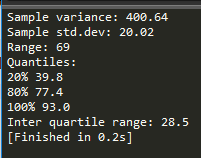The R code for dispersion (variance, standard deviation, range, quantiles, and IQR) is as follows:

```game_points <- c(35,56,43,59,63,79,35,41,64,43,93,60,77,24,82)
dt_var = var(game_points); print(round(dt_var,2))
dt_std = sd(game_points); print(round(dt_std,2))
range_val<-function(x) return(diff(range(x)))
dt_range = range_val(game_points); print(dt_range)
dt_quantile = quantile(game_points,probs = c(0.2,0.8,1.0)); print(dt_quantile)
dt_iqr = IQR(game_points); print(dt_iqr)```
• Hypothesis testing: This is the process of making inferences about the overall population by conducting some statistical tests on a sample. Null and alternate hypotheses are ways to validate whether an assumption is statistically significant or not.
• P-value: The probability of obtaining a test statistic result is at least as extreme as the one that was actually observed, assuming that the null hypothesis is true (usually in modeling, against each independent variable, a p-value less than 0.05 is considered significant and greater than 0.05 is considered insignificant; nonetheless, these values and definitions may change with respect to context).

The steps involved in hypothesis testing are as follows:

1. Assume a null hypothesis (usually no difference, no significance, and so on; a null hypothesis always tries to assume that there is no anomaly pattern and is always homogeneous, and so on).
2. Collect the sample.
3. Calculate test statistics from the sample in order to verify whether the hypothesis is statistically significant or not.
4. Decide either to accept or reject the null hypothesis based on the test statistic.
• Example of hypothesis testing: A chocolate manufacturer who is also your friend claims that all chocolates produced from his factory weigh at least 1,000 g and you have got a funny feeling that it might not be true; you both collected a sample of 30 chocolates and found that the average chocolate weight as 990 g with sample standard deviation as 12.5 g. Given the 0.05 significance level, can we reject the claim made by your friend?

The null hypothesis is that μ0 ≥ 1000 (all chocolates weigh more than 1,000 g).

Collected sample: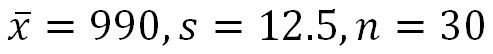Calculate test statistic: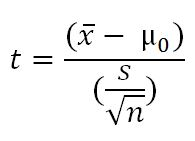t = (990 - 1000) / (12.5/sqrt(30)) = - 4.3818

Critical t value from t tables = t0.05, 30 = 1.699 => - t0.05, 30 = -1.699

P-value = 7.03 e-05

Test statistic is -4.3818, which is less than the critical value of -1.699. Hence, we can reject the null hypothesis (your friend's claim) that the mean weight of a chocolate is above 1,000 g.

Also, another way of deciding the claim is by using the p-value. A p-value less than 0.05 means both claimed values and distribution mean values are significantly different, hence we can reject the null hypothesis: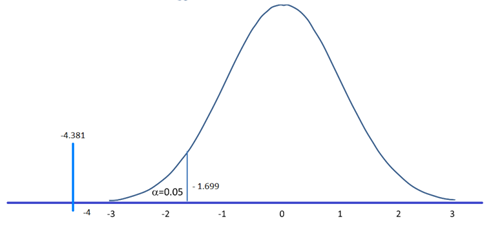The Python code is as follows:

```>>> from scipy import stats
>>> xbar = 990; mu0 = 1000; s = 12.5; n = 30

# Test Statistic
>>> t_smple  = (xbar-mu0)/(s/np.sqrt(float(n))); print ("Test Statistic:",round(t_smple,2))

# Critical value from t-table
>>> alpha = 0.05
>>> t_alpha = stats.t.ppf(alpha,n-1); print ("Critical value from t-table:",round(t_alpha,3))

#Lower tail p-value from t-table
>>> p_val = stats.t.sf(np.abs(t_smple), n-1); print ("Lower tail p-value from t-table", p_val) ```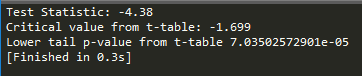The R code for T-distribution is as follows:

```xbar = 990; mu0 = 1000; s = 12.5 ; n = 30
t_smple = (xbar - mu0)/(s/sqrt(n));print (round(t_smple,2))

alpha = 0.05
t_alpha = qt(alpha,df= n-1);print (round(t_alpha,3))

p_val = pt(t_smple,df = n-1);print (p_val)```
• Type I and II error: Hypothesis testing is usually done on the samples rather than the entire population, due to the practical constraints of available resources to collect all the available data. However, performing inferences about the population from samples comes with its own costs, such as rejecting good results or accepting false results, not to mention separately, when increases in sample size lead to minimizing type I and II errors:
• Type I error: Rejecting a null hypothesis when it is true
• Type II error: Accepting a null hypothesis when it is false
• Normal distribution: This is very important in statistics because of the central limit theorem, which states that the population of all possible samples of size n from a population with mean μ and variance σ2 approaches a normal distribution: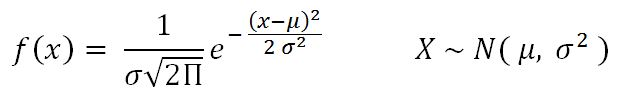Example: Assume that the test scores of an entrance exam fit a normal distribution. Furthermore, the mean test score is 52 and the standard deviation is 16.3. What is the percentage of students scoring 67 or more in the exam?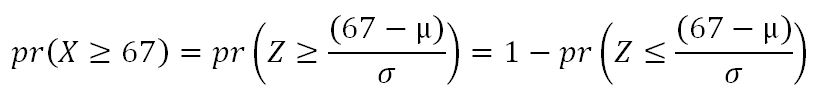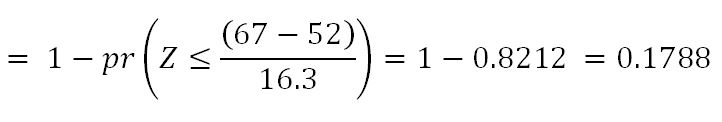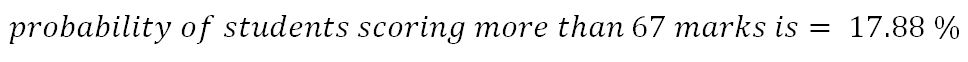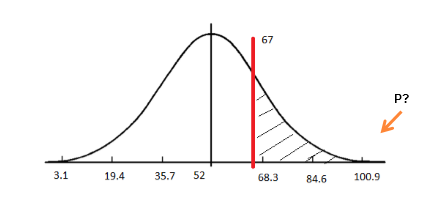The Python code is as follows:

```>>> from scipy import stats
>>> xbar = 67; mu0 = 52; s = 16.3

# Calculating z-score
>>> z = (67-52)/16.3

# Calculating probability under the curve
>>> p_val = 1- stats.norm.cdf(z)
>>> print ("Prob. to score more than 67 is ",round(p_val*100,2),"%")```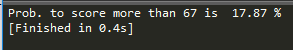The R code for normal distribution is as follows:

```xbar = 67; mu0 = 52; s = 16.3
pr = 1- pnorm(67, mean=52, sd=16.3)
print(paste("Prob. to score more than 67 is ",round(pr*100,2),"%"))```
• Chi-square: This test of independence is one of the most basic and common hypothesis tests in the statistical analysis of categorical data. Given two categorical random variables X and Y, the chi-square test of independence determines whether or not there exists a statistical dependence between them.

The test is usually performed by calculating χ2 from the data and χ2 with (m-1, n-1) degrees from the table. A decision is made as to whether both variables are independent based on the actual value and table value, whichever is higher: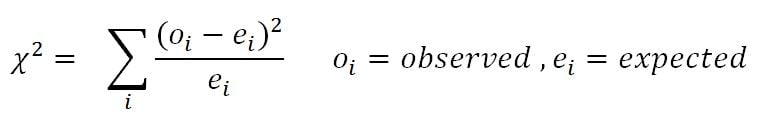Example: In the following table, calculate whether the smoking habit has an impact on exercise behavior: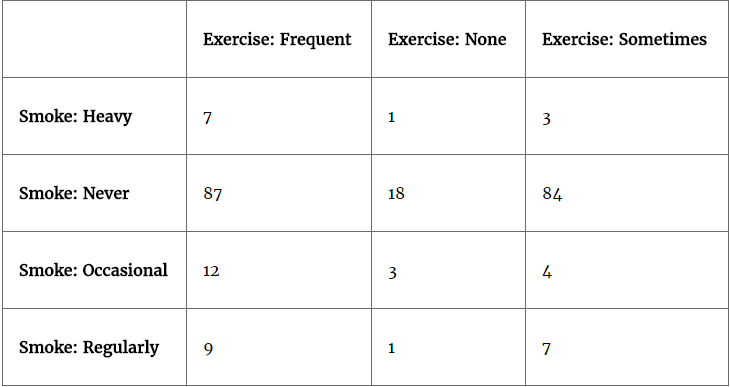The Python code is as follows:

```>>> import pandas as pd
>>> from scipy import stats

# Tabulating 2 variables with row & column variables respectively
>>> survey_tab = pd.crosstab(survey.Smoke, survey.Exer, margins = True)```

While creating a table using the `crosstab` function, we will obtain both row and column totals fields extra. However, in order to create the observed table, we need to extract the variables part and ignore the totals:

```# Creating observed table for analysis
>>> observed = survey_tab.ix[0:4,0:3] ```

The `chi2_contingency` function in the stats package uses the observed table and subsequently calculates its expected table, followed by calculating the p-value in order to check whether two variables are dependent or not. If p-value < 0.05, there is a strong dependency between two variables, whereas if p-value > 0.05, there is no dependency between the variables:

```>>> contg = stats.chi2_contingency(observed= observed)
>>> p_value = round(contg,3)
>>> print ("P-value is: ",p_value)```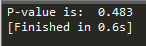The p-value is `0.483`, which means there is no dependency between the smoking habit and exercise behavior.

The R code for chi-square is as follows:

```survey = read.csv("survey.csv",header=TRUE)
tbl = table(survey\$Smoke,survey\$Exer)
p_val = chisq.test(tbl)```
• ANOVA: Analyzing variance tests the hypothesis that the means of two or more populations are equal. ANOVAs assess the importance of one or more factors by comparing the response variable means at the different factor levels. The null hypothesis states that all population means are equal while the alternative hypothesis states that at least one is different.

Example: A fertilizer company developed three new types of universal fertilizers after research that can be utilized to grow any type of crop. In order to find out whether all three have a similar crop yield, they randomly chose six crop types in the study. In accordance with the randomized block design, each crop type will be tested with all three types of fertilizer separately. The following table represents the yield in g/m2. At the 0.05 level of significance, test whether the mean yields for the three new types of fertilizers are all equal:

 Fertilizer 1 Fertilizer 2 Fertilizer 3 62 54 48 62 56 62 90 58 92 42 36 96 84 72 92 64 34 80

The Python code is as follows:

```>>> import pandas as pd
>>> from scipy import stats

Calculating one-way ANOVA using the `stats` package:

```>>> one_way_anova = stats.f_oneway(fetilizers["fertilizer1"], fetilizers["fertilizer2"], fetilizers["fertilizer3"])

>>> print ("Statistic :", round(one_way_anova,2),", p-value :",round(one_way_anova,3))```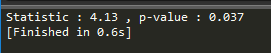Result: The p-value did come as less than 0.05, hence we can reject the null hypothesis that the mean crop yields of the fertilizers are equal. Fertilizers make a significant difference to crops.

The R code for ANOVA is as follows:

```fetilizers = read.csv("fetilizers.csv",header=TRUE)
r = c(t(as.matrix(fetilizers)))
f = c("fertilizer1","fertilizer2","fertilizer3")
k = 3; n = 6
tm = gl(k,1,n*k,factor(f))
blk = gl(n,k,k*n)
av = aov(r ~ tm + blk)
smry = summary(av)```
• Confusion matrix: This is the matrix of the actual versus the predicted. This concept is better explained with the example of cancer prediction using the model: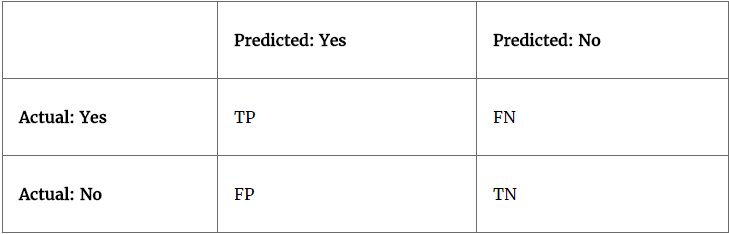Some terms used in a confusion matrix are:

• True positives (TPs): True positives are cases when we predict the disease as yes when the patient actually does have the disease.
• True negatives (TNs): Cases when we predict the disease as no when the patient actually does not have the disease.
• False positives (FPs): When we predict the disease as yes when the patient actually does not have the disease. FPs are also considered to be type I errors.
• False negatives (FNs): When we predict the disease as no when the patient actually does have the disease. FNs are also considered to be type II errors.
• Precision (P): When yes is predicted, how often is it correct?

(TP/TP+FP)

• Recall (R)/sensitivity/true positive rate: Among the actual yeses, what fraction was predicted as yes?

(TP/TP+FN)

• F1 score (F1): This is the harmonic mean of the precision and recall. Multiplying the constant of 2 scales the score to 1 when both precision and recall are 1: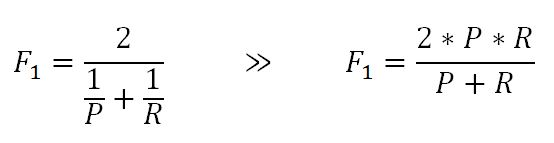• Specificity: Among the actual nos, what fraction was predicted as no? Also equivalent to 1- false positive rate:

(TN/TN+FP)

• Area under curve (ROC): Receiver operating characteristic curve is used to plot between true positive rate (TPR) and false positive rate (FPR), also known as a sensitivity and 1- specificity graph: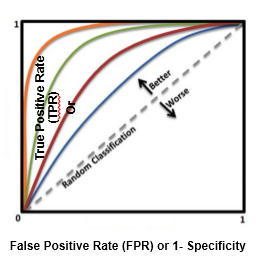Area under curve is utilized for setting the threshold of cut-off probability to classify the predicted probability into various classes; we will be covering how this method works in upcoming chapters.

• Observation and performance window: In statistical modeling, the model tries to predict the event in advance rather than at the moment, so that some buffer time will exist to work on corrective actions. For example, a question from a credit card company would be, for example, what is the probability that a particular customer will default in the coming 12-month period? So that I can call him and offer any discounts or develop my collection strategies accordingly.

In order to answer this question, a probability of default model (or behavioral scorecard in technical terms) needs to be developed by using independent variables from the past 24 months and a dependent variable from the next 12 months. After preparing data with X and Y variables, it will be split into 70 percent - 30 percent as train and test data randomly; this method is called in-time validation as both train and test samples are from the same time period: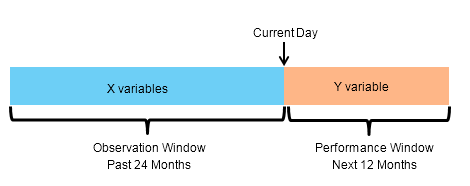• In-time and out-of-time validation: In-time validation implies obtaining both a training and testing dataset from the same period of time, whereas out-of-time validation implies training and testing datasets drawn from different time periods. Usually, the model performs worse in out-of-time validation rather than in-time due to the obvious reason that the characteristics of the train and test datasets might differ.
• R-squared (coefficient of determination): This is the measure of the percentage of the response variable variation that is explained by a model. It also a measure of how well the model minimizes error compared with just utilizing the mean as an estimate. In some extreme cases, R-squared can have a value less than zero also, which means the predicted values from the model perform worse than just taking the simple mean as a prediction for all the observations. We will study this parameter in detail in upcoming chapters: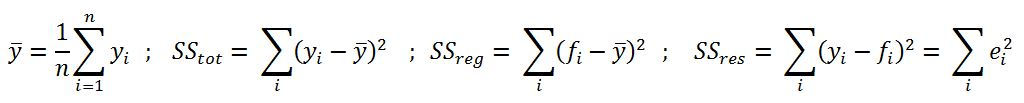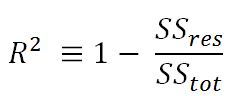• Adjusted R-squared: The explanation of the adjusted R-squared statistic is almost the same as R-squared but it penalizes the R-squared value if extra variables without a strong correlation are included in the model: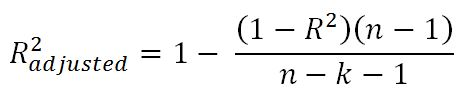Here, R2 = sample R-squared value, n = sample size, k = number of predictors (or) variables.

Adjusted R-squared value is the key metric in evaluating the quality of linear regressions. Any linear regression model having the value of R2 adjusted >= 0.7 is considered as a good enough model to implement.

Example: The R-squared value of a sample is 0.5, with a sample size of 50 and the independent variables are 10 in number. Calculated adjusted R-squared: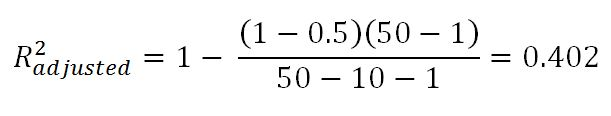• Maximum likelihood estimate (MLE): This is estimating the parameter values of a statistical model (logistic regression, to be precise) by finding the parameter values that maximize the likelihood of making the observations. We will cover this method in more depth in Chapter 3, Logistic Regression Versus Random Forest.
• Akaike information criteria (AIC): This is used in logistic regression, which is similar to the principle of adjusted R-square for linear regression. It measures the relative quality of a model for a given set of data: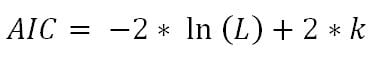Here, k = number of predictors or variables

The idea of AIC is to penalize the objective function if extra variables without strong predictive abilities are included in the model. This is a kind of regularization in logistic regression.

• Entropy: This comes from information theory and is the measure of impurity in the data. If the sample is completely homogeneous, the entropy is zero and if the sample is equally divided, it has an entropy of 1. In decision trees, the predictor with the most heterogeneousness will be considered nearest to the root node to classify given data into classes in a greedy mode. We will cover this topic in more depth in Chapter 4, Tree-Based Machine Learning Models:Here, n = number of classes. Entropy is maximal at the middle, with the value of 1 and minimal at the extremes as 0. A low value of entropy is desirable as it will segregate classes better: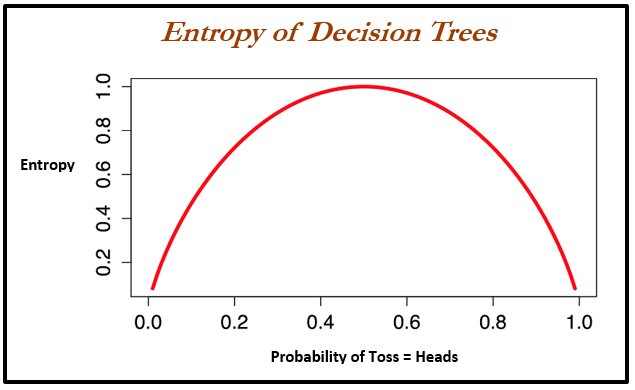Example: Given two types of coin in which the first one is a fair one (1/2 head and 1/2 tail probabilities) and the other is a biased one (1/3 head and 2/3 tail probabilities), calculate the entropy for both and justify which one is better with respect to modeling: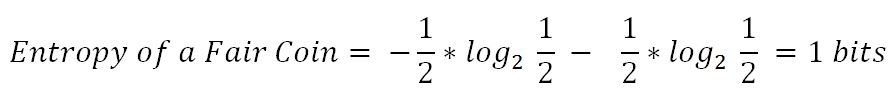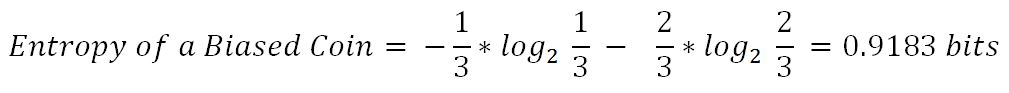From both values, the decision tree algorithm chooses the biased coin rather than the fair coin as an observation splitter due to the fact the value of entropy is less.

• Information gain: This is the expected reduction in entropy caused by partitioning the examples according to a given attribute. The idea is to start with mixed classes and to keep partitioning until each node reaches its observations of the purest class. At every stage, the variable with maximum information gain is chosen in greedy fashion:

Information gain = Entropy of parent - sum (weighted % * Entropy of child)

Weighted % = Number of observations in particular child / sum (observations in all child nodes)

• Gini: Gini impurity is a measure of misclassification, which applies in a multiclass classifier context. Gini works almost the same as entropy, except Gini is faster to calculate: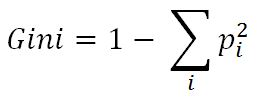Here, i = number of classes. The similarity between Gini and entropy is shown as follows: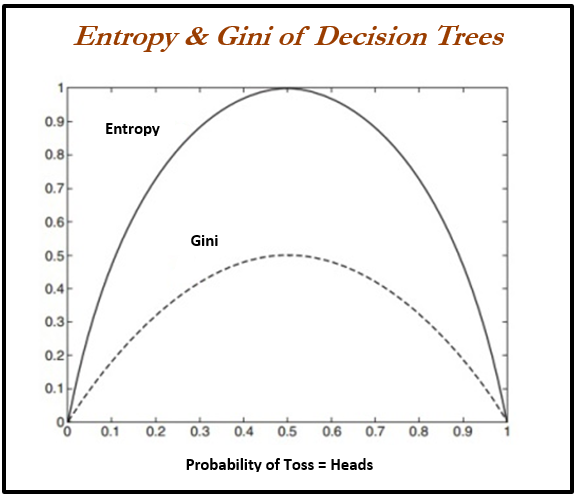Every model has both bias and variance error components in addition to white noise. Bias and variance are inversely related to each other; while trying to reduce one component, the other component of the model will increase. The true art lies in creating a good fit by balancing both. The ideal model will have both low bias and low variance.

Errors from the bias component come from erroneous assumptions in the underlying learning algorithm. High bias can cause an algorithm to miss the relevant relations between features and target outputs; this phenomenon causes an underfitting problem.

On the other hand, errors from the variance component come from sensitivity to change in the fit of the model, even a small change in training data; high variance can cause an overfitting problem: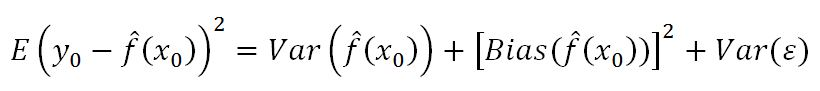An example of a high bias model is logistic or linear regression, in which the fit of the model is merely a straight line and may have a high error component due to the fact that a linear model could not approximate underlying data well.

An example of a high variance model is a decision tree, in which the model may create too much wiggly curve as a fit, in which even a small change in training data will cause a drastic change in the fit of the curve.

At the moment, state-of-the-art models are utilizing high variance models such as decision trees and performing ensemble on top of them to reduce the errors caused by high variance and at the same time not compromising on increases in errors due to the bias component. The best example of this category is random forest, in which many decision trees will be grown independently and ensemble in order to come up with the best fit; we will cover this in upcoming chapters: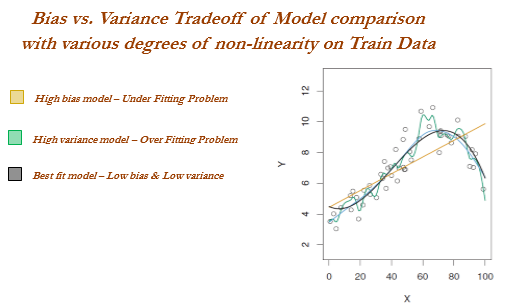### Train and test data

In practice, data usually will be split randomly 70-30 or 80-20 into train and test datasets respectively in statistical modeling, in which training data utilized for building the model and its effectiveness will be checked on test data: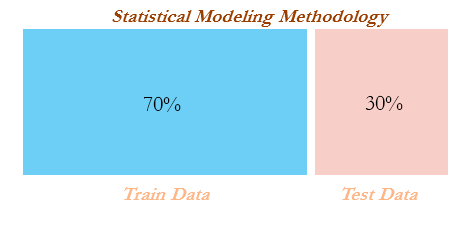In the following code, we split the original data into train and test data by 70 percent - 30 percent. An important point to consider here is that we set the seed values for random numbers in order to repeat the random sampling every time we create the same observations in training and testing data. Repeatability is very much needed in order to reproduce the results:

```# Train & Test split
>>> import pandas as pd
>>> from sklearn.model_selection import train_test_split

In the following code, `train size` is `0.7`, which means 70 percent of the data should be split into the training dataset and the remaining 30% should be in the testing dataset. Random state is seed in this process of generating pseudo-random numbers, which makes the results reproducible by splitting the exact same observations while running every time:

`>>> train_data,test_data = train_test_split(original_data,train_size = 0.7,random_state=42)`

The R code for the train and test split for statistical modeling is as follows:

```full_data = read.csv("mtcars.csv",header=TRUE)
set.seed(123)
numrow = nrow(full_data)
trnind = sample(1:numrow,size = as.integer(0.7*numrow))
train_data = full_data[trnind,]
test_data = full_data[-trnind,]```

## Machine learning terminology for model building and validation

There seems to be an analogy between statistical modeling and machine learning that we will cover in subsequent chapters in depth. However, a quick view has been provided as follows: in statistical modeling, linear regression with two independent variables is trying to fit the best plane with the least errors, whereas in machine learning independent variables have been converted into the square of error terms (squaring ensures the function will become convex, which enhances faster convergence and also ensures a global optimum) and optimized based on coefficient values rather than independent variables: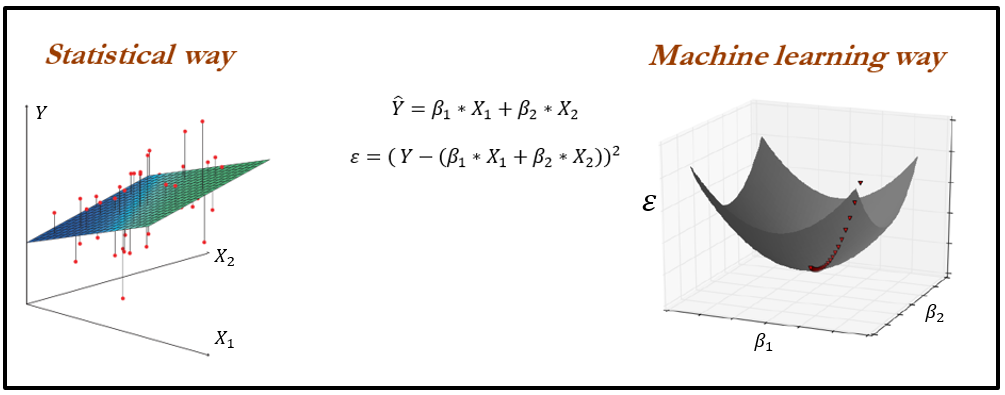Machine learning utilizes optimization for tuning all the parameters of various algorithms. Hence, it is a good idea to know some basics about optimization.

Before stepping into gradient descent, the introduction of convex and non-convex functions is very helpful. Convex functions are functions in which a line drawn between any two random points on the function also lies within the function, whereas this isn't true for non-convex functions. It is important to know whether the function is convex or non-convex due to the fact that in convex functions, the local optimum is also the global optimum, whereas for non-convex functions, the local optimum does not guarantee the global optimum: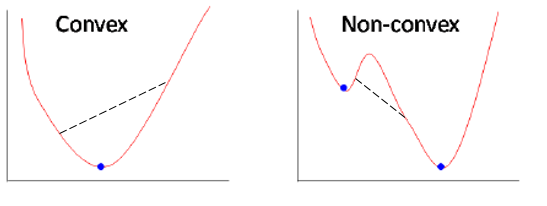Does it seem like a tough problem? One turnaround could be to initiate a search process at different random locations; by doing so, it usually converges to the global optimum:

• Gradient descent: This is a way to minimize the objective function J(Θ) parameterized by the model's parameter Θε Rd by updating the parameters in the opposite direction to the gradient of the objective function with respect to the parameters. The learning rate determines the size of steps taken to reach the minimum.
• Full batch gradient descent (all training observations considered in each and every iteration): In full batch gradient descent, all the observations are considered for each and every iteration; this methodology takes a lot of memory and will be slow as well. Also, in practice, we do not need to have all the observations to update the weights. Nonetheless, this method provides the best way of updating parameters with less noise at the expense of huge computation.
• Stochastic gradient descent (one observation per iteration): This method updates weights by taking one observation at each stage of iteration. This method provides the quickest way of traversing weights; however, a lot of noise is involved while converging.
• Mini batch gradient descent (about 30 training observations or more for each and every iteration): This is a trade-off between huge computational costs and a quick method of updating weights. In this method, at each iteration, about 30 observations will be selected at random and gradients calculated to update the model weights. Here, a question many can ask is, why the minimum 30 and not any other number? If we look into statistical basics, 30 observations required to be considering in order approximating sample as a population. However, even 40, 50, and so on will also do well in batch size selection. Nonetheless, a practitioner needs to change the batch size and verify the results, to determine at what value the model is producing the optimum results: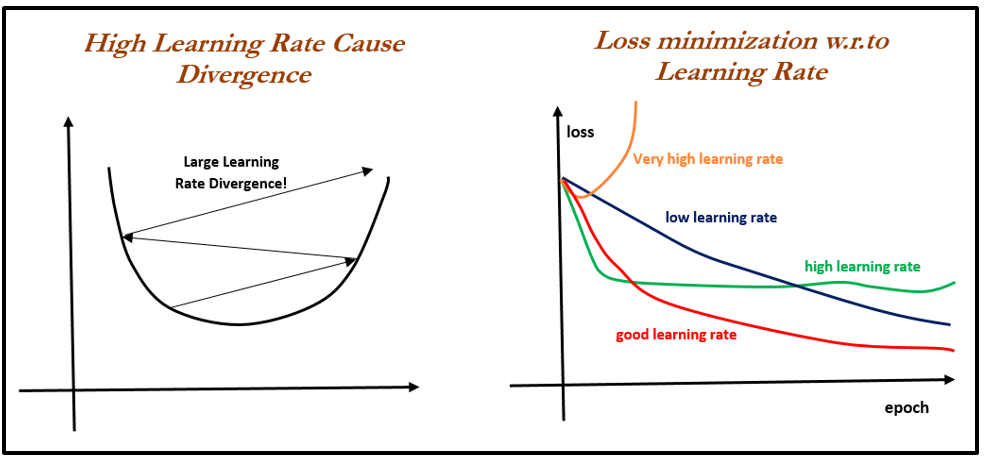### Linear regression versus gradient descent

In the following code, a comparison has been made between applying linear regression in a statistical way and gradient descent in a machine learning way on the same dataset:

```>>> import numpy as np
>>> import pandas as pd```

The following code describes reading data using a pandas DataFrame:

`>>> train_data = pd.read_csv("mtcars.csv") `

Converting DataFrame variables into NumPy arrays in order to process them in scikit learn packages, as scikit-learn is built on NumPy arrays itself, is shown next:

```>>> X = np.array(train_data["hp"])  ; y = np.array(train_data["mpg"])
>>> X = X.reshape(32,1); y = y.reshape(32,1)```

Importing linear regression from the scikit-learn package; this works on the least squares method:

```>>> from sklearn.linear_model import LinearRegression
>>> model = LinearRegression(fit_intercept = True)```

Fitting a linear regression model on the data and display intercept and coefficient of single variable (`hp` variable):

```>>> model.fit(X,y)
>>> print ("Linear Regression Results" )
>>> print ("Intercept",model.intercept_ ,"Coefficient", model.coef_)```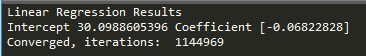Now we will apply gradient descent from scratch; in future chapters, we can use the scikit-learn built-in modules rather than doing it from first principles. However, here, an illustration has been provided on the internal workings of the optimization method on which the whole machine learning has been built.

Defining the gradient descent function `gradient_descent` with the following:

• `x`: Independent variable.
• `y`: Dependent variable.
• `learn_rate`: Learning rate with which gradients are updated; too low causes slower convergence and too high causes overshooting of gradients.
• `batch_size`: Number of observations considered at each iteration for updating gradients; a high number causes a lower number of iterations and a lower number causes an erratic decrease in errors. Ideally, the batch size should be a minimum value of 30 due to statistical significance. However, various settings need to be tried to check which one is better.
• `max_iter`: Maximum number of iteration, beyond which the algorithm will get auto-terminated:
```>>> def gradient_descent(x, y,learn_rate, conv_threshold,batch_size, max_iter):
...    converged = False
...    iter = 0
...    m = batch_size
...    t0 = np.random.random(x.shape)
...    t1 = np.random.random(x.shape)```

### Note

`Mean square error calculation`Squaring of error has been performed to create the convex function, which has nice convergence properties:`... MSE = (sum([(t0 + t1*x[i] - y[i])**2 for i in range(m)])/ m)`

The following code states, run the algorithm until it does not meet the convergence criteria:

```...      while not converged:
...          grad0 = 1.0/m * sum([(t0 + t1*x[i] - y[i]) for i in range(m)])
...          grad1 = 1.0/m * sum([(t0 + t1*x[i] - y[i])*x[i] for i in range(m)])
...          temp0 = t0 - learn_rate * grad0
...          temp1 = t1 - learn_rate * grad1
...          t0 = temp0
...          t1 = temp1```

Calculate a new error with updated parameters, in order to check whether the new error changed more than the predefined convergence threshold value; otherwise, stop the iterations and return parameters:

```...          MSE_New = (sum( [ (t0 + t1*x[i] - y[i])**2 for i in range(m)] ) / m)
...          if abs(MSE - MSE_New ) <= conv_threshold:
...              print 'Converged, iterations: ', iter
...              converged = True
...          MSE = MSE_New
...          iter += 1
...          if iter == max_iter:
...              print 'Max interactions reached'
...              converged = True
...          return t0,t1```

The following code describes running the gradient descent function with defined values. Learn rate = 0.0003, convergence threshold = 1e-8, batch size = 32, maximum number of iteration = 1500000:

```>>> if __name__ == '__main__':
...      Inter, Coeff = gradient_descent(x = X,y = y,learn_rate=0.00003 , conv_threshold = 1e-8, batch_size=32,max_iter=1500000)
...      print (('Intercept = %s Coefficient = %s') %(Inter, Coeff))```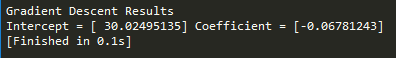The R code for linear regression versus gradient descent is as follows:

```# Linear Regression
model <- lm(mpg ~ hp, data = train_data)
print (coef(model))

gradDesc <- function(x, y, learn_rate, conv_threshold, batch_size, max_iter) {
m <- runif(1, 0, 1)
c <- runif(1, 0, 1)
ypred <- m * x + c
MSE <- sum((y - ypred) ^ 2) / batch_size

converged = F
iterations = 0

while(converged == F) {
m_new <- m - learn_rate * ((1 / batch_size) * (sum((ypred - y) * x)))
c_new <- c - learn_rate * ((1 / batch_size) * (sum(ypred - y)))

m <- m_new
c <- c_new
ypred <- m * x + c
MSE_new <- sum((y - ypred) ^ 2) / batch_size

if(MSE - MSE_new <= conv_threshold) {
converged = T
return(paste("Iterations:",iterations,"Optimal intercept:", c, "Optimal slope:", m))
}
iterations = iterations + 1

if(iterations > max_iter) {
converged = T
return(paste("Iterations:",iterations,"Optimal intercept:", c, "Optimal slope:", m))
}
MSE = MSE_new
}
}
gradDesc(x = train_data\$hp,y =  train_data\$mpg, learn_rate = 0.00003, conv_threshold = 1e-8, batch_size = 32, max_iter = 1500000)```

### Machine learning losses

The loss function or cost function in machine learning is a function that maps the values of variables onto a real number intuitively representing some cost associated with the variable values. Optimization methods are applied to minimize the loss function by changing the parameter values, which is the central theme of machine learning.

Zero-one loss is L0-1 = 1 (m <= 0); in zero-one loss, value of loss is 0 for m >= 0 whereas 1 for m < 0. The difficult part with this loss is it is not differentiable, non-convex, and also NP-hard. Hence, in order to make optimization feasible and solvable, these losses are replaced by different surrogate losses for different problems.

Surrogate losses used for machine learning in place of zero-one loss are given as follows. The zero-one loss is not differentiable, hence approximated losses are being used instead:

• Squared loss (for regression)
• Hinge loss (SVM)
• Logistic/log loss (logistic regression)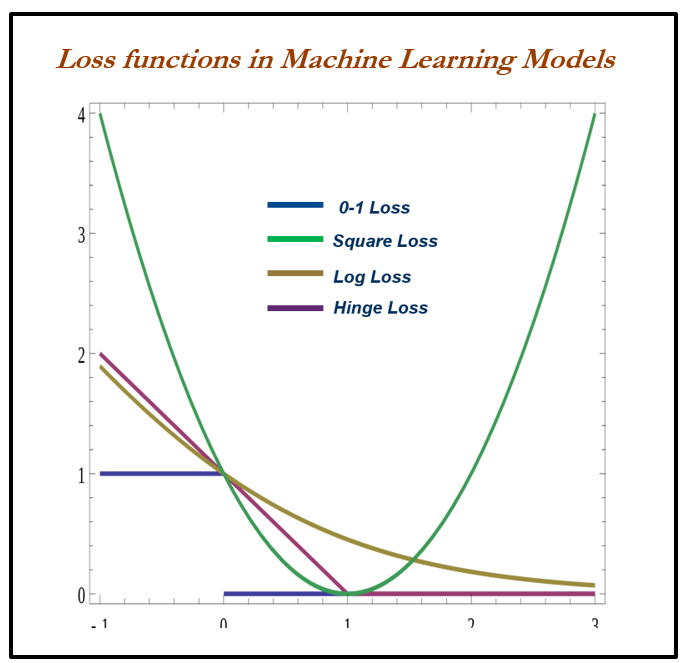Some loss functions are as follows: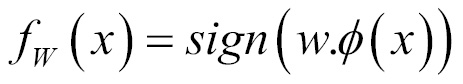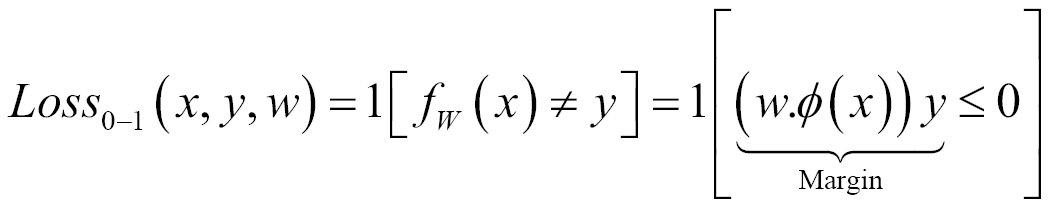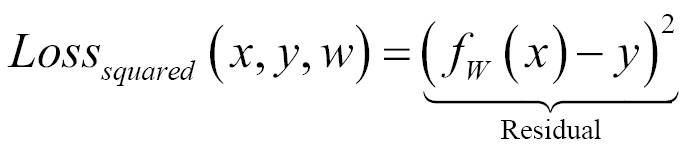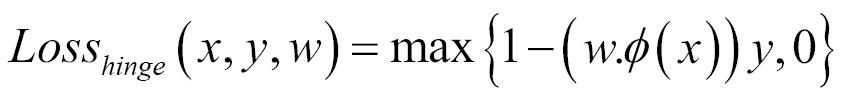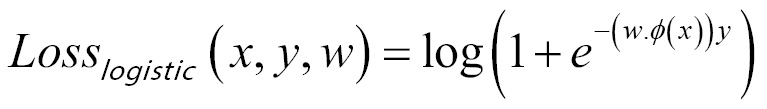### When to stop tuning machine learning models

When to stop tuning the hyperparameters in a machine learning model is a million-dollar question. This problem can be mostly solved by keeping tabs on training and testing errors. While increasing the complexity of a model, the following stages occur:

• Stage 1: Underfitting stage - high train and high test errors (or low train and low test accuracy)
• Stage 2: Good fit stage (ideal scenario) - low train and low test errors (or high train and high test accuracy)
• Stage 3: Overfitting stage - low train and high test errors (or high train and low test accuracy)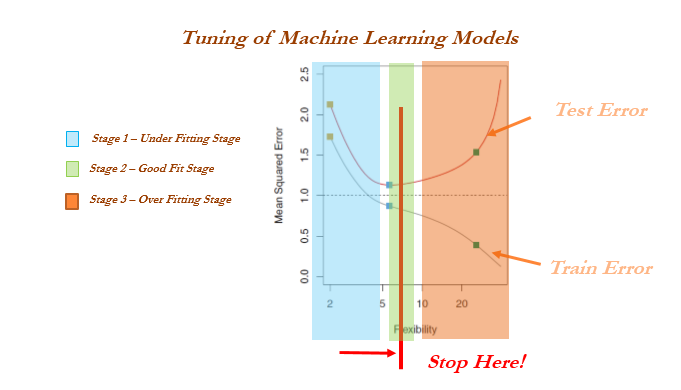### Train, validation, and test data

Cross-validation is not popular in the statistical modeling world for many reasons; statistical models are linear in nature and robust, and do not have a high variance/overfitting problem. Hence, the model fit will remain the same either on train or test data, which does not hold true in the machine learning world. Also, in statistical modeling, lots of tests are performed at the individual parameter level apart from aggregated metrics, whereas in machine learning we do not have visibility at the individual parameter level: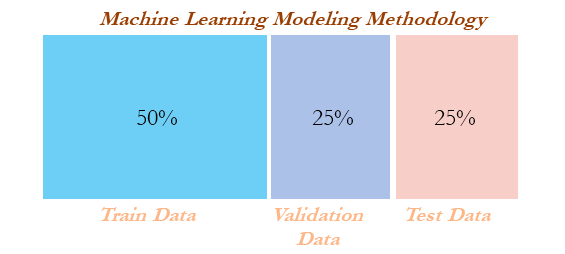In the following code, both the R and Python implementation has been provided. If none of the percentages are provided, the default parameters are 50 percent for train data, 25 percent for validation data, and 25 percent for the remaining test data.

Python implementation has only one train and test split functionality, hence we have used it twice and also used the number of observations to split rather than the percentage (as shown in the previous train and test split example). Hence, a customized function is needed to split into three datasets:

```>>> import pandas as pd
>>> from sklearn.model_selection import train_test_split

>>> def data_split(dat,trf = 0.5,vlf=0.25,tsf = 0.25):
...      nrows = dat.shape
...      trnr = int(nrows*trf)
...      vlnr = int(nrows*vlf)    ```

The following Python code splits the data into training and the remaining data. The remaining data will be further split into validation and test datasets:

```...      tr_data,rmng = train_test_split(dat,train_size = trnr,random_state=42)
...      vl_data, ts_data = train_test_split(rmng,train_size = vlnr,random_state=45)
...      return (tr_data,vl_data,ts_data)```

Implementation of the split function on the original data to create three datasets (by 50 percent, 25 percent, and 25 percent splits) is as follows:

`>>> train_data, validation_data, test_data = data_split (original_data ,trf=0.5, vlf=0.25,tsf=0.25)`

The R code for the train, validation, and test split is as follows:

```# Train Validation & Test samples
trvaltest <- function(dat,prop = c(0.5,0.25,0.25)){
nrw = nrow(dat)
trnr = as.integer(nrw *prop)
vlnr = as.integer(nrw*prop)
set.seed(123)
trni = sample(1:nrow(dat),trnr)
trndata = dat[trni,]
rmng = dat[-trni,]
vlni = sample(1:nrow(rmng),vlnr)
valdata = rmng[vlni,]
tstdata = rmng[-vlni,]
mylist = list("trn" = trndata,"val"= valdata,"tst" = tstdata)
return(mylist)
}
outdata = trvaltest(mtcars,prop = c(0.5,0.25,0.25))
train_data = outdata\$trn; valid_data = outdata\$val; test_data = outdata\$tst```

### Cross-validation

Cross-validation is another way of ensuring robustness in the model at the expense of computation. In the ordinary modeling methodology, a model is developed on train data and evaluated on test data. In some extreme cases, train and test might not have been homogeneously selected and some unseen extreme cases might appear in the test data, which will drag down the performance of the model.

On the other hand, in cross-validation methodology, data was divided into equal parts and training performed on all the other parts of the data except one part, on which performance will be evaluated. This process repeated as many parts user has chosen.

Example: In five-fold cross-validation, data will be divided into five parts, subsequently trained on four parts of the data, and tested on the one part of the data. This process will run five times, in order to cover all points in the data. Finally, the error calculated will be the average of all the errors: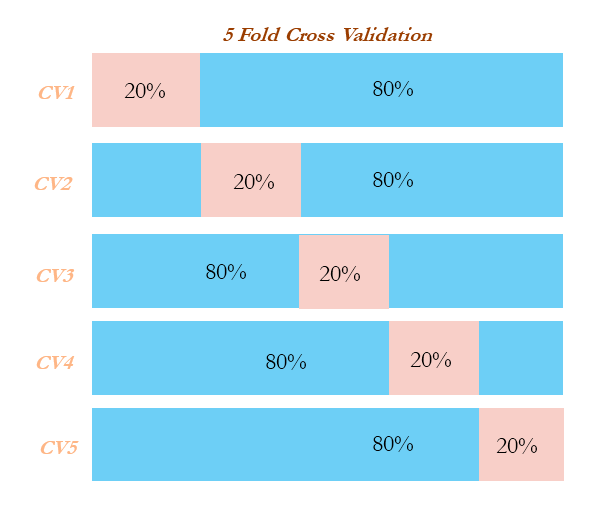### Grid search

Grid search in machine learning is a popular way to tune the hyperparameters of the model in order to find the best combination for determining the best fit: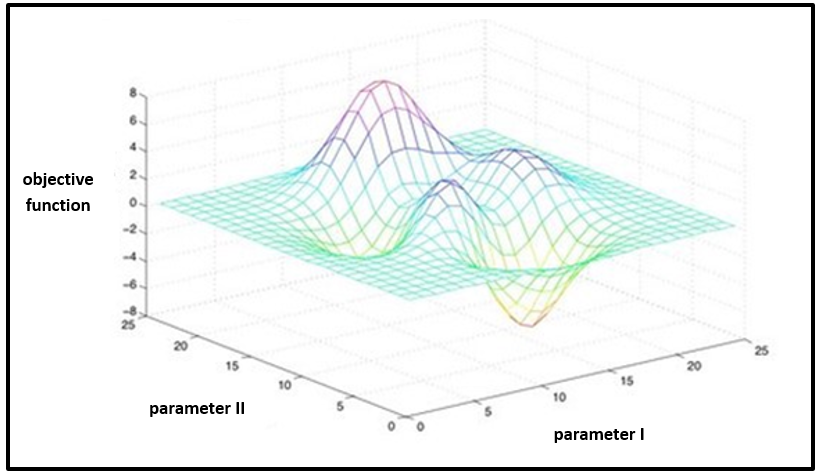In the following code, implementation has been performed to determine whether a particular user will click an ad or not. Grid search has been implemented using a decision tree classifier for classification purposes. Tuning parameters are the depth of the tree, the minimum number of observations in terminal node, and the minimum number of observations required to perform the node split:

```# Grid search
>>> import pandas as pd
>>> from sklearn.tree import DecisionTreeClassifier
>>> from sklearn.model_selection import train_test_split
>>> from sklearn.metrics import classification_report,confusion_matrix,accuracy_score
>>> from sklearn.pipeline import Pipeline
>>> from sklearn.grid_search import GridSearchCV

>>> X_columns = set(input_data.columns.values)
>>> y = input_data[len(input_data.columns.values)-1]
>>> X_columns.remove(len(input_data.columns.values)-1)
>>> X = input_data[list(X_columns)]```

Split the data into train and testing:

`>>> X_train, X_test,y_train,y_test = train_test_split(X,y,train_size = 0.7,random_state=33)`

Create a pipeline to create combinations of variables for the grid search:

```>>> pipeline = Pipeline([
...      ('clf', DecisionTreeClassifier(criterion='entropy')) ])```

Combinations to explore are given as parameters in Python dictionary format:

```>>> parameters = {
...      'clf__max_depth': (50,100,150),
...      'clf__min_samples_split': (2, 3),
...      'clf__min_samples_leaf': (1, 2, 3)}```

The `n_jobs` field is for selecting the number of cores in a computer; `-1` means it uses all the cores in the computer. The scoring methodology is accuracy, in which many other options can be chosen, such as `precision`, `recall`, and `f1`:

```>>> grid_search = GridSearchCV(pipeline, parameters, n_jobs=-1, verbose=1, scoring='accuracy')
>>> grid_search.fit(X_train, y_train) ```

Predict using the best parameters of grid search:

`>>> y_pred = grid_search.predict(X_test)`

The output is as follows:

```>>> print ('\n Best score: \n', grid_search.best_score_)
>>> print ('\n Best parameters set: \n')
>>> best_parameters = grid_search.best_estimator_.get_params()
>>> for param_name in sorted(parameters.keys()):
>>>     print ('\t%s: %r' % (param_name, best_parameters[param_name]))
>>> print ("\n Confusion Matrix on Test data \n",confusion_matrix(y_test,y_pred))
>>> print ("\n Test Accuracy \n",accuracy_score(y_test,y_pred))
>>> print ("\nPrecision Recall f1 table \n",classification_report(y_test, y_pred))```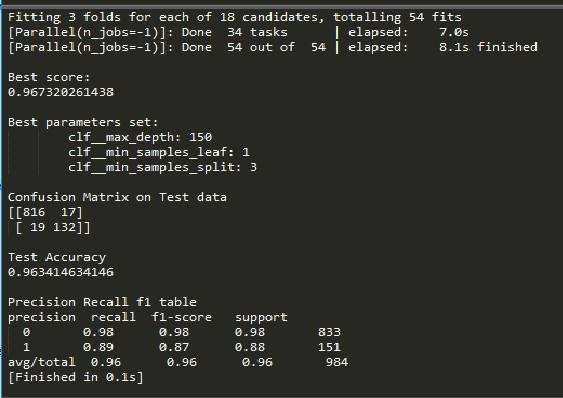The R code for grid searches on decision trees is as follows:

```# Grid Search on Decision Trees
library(rpart)
input_data\$V1559 = as.factor(input_data\$V1559)
set.seed(123)
numrow = nrow(input_data)
trnind = sample(1:numrow,size = as.integer(0.7*numrow))

train_data = input_data[trnind,];test_data = input_data[-trnind,]
minspset = c(2,3);minobset = c(1,2,3)
initacc = 0

for (minsp in minspset){
for (minob in minobset){
tr_fit = rpart(V1559 ~.,data = train_data,method = "class",minsplit = minsp, minbucket = minob)
tr_predt = predict(tr_fit,newdata = train_data,type = "class")
tble = table(tr_predt,train_data\$V1559)
acc = (tble[1,1]+tble[2,2])/sum(tble)
acc
if (acc > initacc){
tr_predtst = predict(tr_fit,newdata = test_data,type = "class")
tblet = table(test_data\$V1559,tr_predtst)
acct = (tblet[1,1]+tblet[2,2])/sum(tblet)
acct
print(paste("Best Score"))
print( paste("Train Accuracy ",round(acc,3),"Test Accuracy",round(acct,3)))
print( paste(" Min split ",minsp," Min obs per node ",minob))
print(paste("Confusion matrix on test data"))
print(tblet)
precsn_0 = (tblet[1,1])/(tblet[1,1]+tblet[2,1])
precsn_1 = (tblet[2,2])/(tblet[1,2]+tblet[2,2])
print(paste("Precision_0: ",round(precsn_0,3),"Precision_1: ",round(precsn_1,3)))
rcall_0 = (tblet[1,1])/(tblet[1,1]+tblet[1,2])
rcall_1 = (tblet[2,2])/(tblet[2,1]+tblet[2,2])
print(paste("Recall_0: ",round(rcall_0,3),"Recall_1: ",round(rcall_1,3)))
initacc = acc
}
}
}```

## Machine learning model overview

Machine learning models are classified mainly into supervised, unsupervised, and reinforcement learning methods. We will be covering detailed discussions about each technique in later chapters; here is a very basic summary of them:

• Supervised learning: This is where an instructor provides feedback to a student on whether they have performed well in an examination or not. In which target variable do present and models do get tune to achieve it. Many machine learning methods fall in to this category:
• Classification problems
• Logistic regression
• Lasso and ridge regression
• Decision trees (classification trees)
• Bagging classifier
• Random forest classifier
• SVM classifier
• Recommendation engine
• Regression problems
• Linear regression (lasso and ridge regression)
• Decision trees (regression trees)
• Bagging regressor
• Random forest regressor
• SVM regressor
• Unsupervised learning: Similar to the teacher-student analogy, in which the instructor does not present and provide feedback to the student and who needs to prepare on his/her own. Unsupervised learning does not have as many are in supervised learning:
• Principal component analysis (PCA)
• K-means clustering
• Reinforcement learning: This is the scenario in which multiple decisions need to be taken by an agent prior to reaching the target and it provides a reward, either +1 or -1, rather than notifying how well or how badly the agent performed across the path:
• Markov decision process
• Monte Carlo methods
• Temporal difference learning
• Logistic regression: This is the problem in which outcomes are discrete classes rather than continuous values. For example, a customer will arrive or not, he will purchase the product or not, and so on. In statistical methodology, it uses the maximum likelihood method to calculate the parameter of individual variables. In contrast, in machine learning methodology, log loss will be minimized with respect to β coefficients (also known as weights). Logistic regression has a high bias and a low variance error.
• Linear regression: This is used for the prediction of continuous variables such as customer income and so on. It utilizes error minimization to fit the best possible line in statistical methodology. However, in machine learning methodology, squared loss will be minimized with respect to β coefficients. Linear regression also has a high bias and a low variance error.
• Lasso and ridge regression: This uses regularization to control overfitting issues by applying a penalty on coefficients. In ridge regression, a penalty is applied on the sum of squares of coefficients, whereas in lasso, a penalty is applied on the absolute values of the coefficients. The penalty can be tuned in order to change the dynamics of the model fit. Ridge regression tries to minimize the magnitude of coefficients, whereas lasso tries to eliminate them.
• Decision trees: Recursive binary splitting is applied to split the classes at each level to classify observations to their purest class. The classification error rate is simply the fraction of the training observations in that region that do not belong to the most common class. Decision trees have an overfitting problem due to their high variance in a way to fit; pruning is applied to reduce the overfitting problem by growing the tree completely. Decision trees have low a bias and a high variance error.
• Bagging: This is an ensemble technique applied on decision trees in order to minimize the variance error and at the same time not increase the error component due to bias. In bagging, various samples are selected with a subsample of observations and all variables (columns), subsequently fit individual decision trees independently on each sample and later ensemble the results by taking the maximum vote (in regression cases, the mean of outcomes calculated).
• Random forest: This is similar to bagging except for one difference. In bagging, all the variables/columns are selected for each sample, whereas in random forest a few subcolumns are selected. The reason behind the selection of a few variables rather than all was that during each independent tree sampled, significant variables always came first in the top layer of splitting which makes all the trees look more or less similar and defies the sole purpose of ensemble: that it works better on diversified and independent individual models rather than correlated individual models. Random forest has both low bias and variance errors.
• Boosting: This is a sequential algorithm that applies on weak classifiers such as a decision stump (a one-level decision tree or a tree with one root node and two terminal nodes) to create a strong classifier by ensembling the results. The algorithm starts with equal weights assigned to all the observations, followed by subsequent iterations where more focus was given to misclassified observations by increasing the weight of misclassified observations and decreasing the weight of properly classified observations. In the end, all the individual classifiers were combined to create a strong classifier. Boosting might have an overfitting problem, but by carefully tuning the parameters, we can obtain the best of the self machine learning model.
• Support vector machines (SVMs): This maximizes the margin between classes by fitting the widest possible hyperplane between them. In the case of non-linearly separable classes, it uses kernels to move observations into higher-dimensional space and then separates them linearly with the hyperplane there.
• Recommendation engine: This utilizes a collaborative filtering algorithm to identify high-probability items to its respective users, who have not used it in the past, by considering the tastes of similar users who would be using that particular item. It uses the alternating least squares (ALS) methodology to solve this problem.
• Principal component analysis (PCA): This is a dimensionality reduction technique in which principal components are calculated in place of the original variable. Principal components are determined where the variance in data is maximum; subsequently, the top n components will be taken by covering about 80 percent of variance and will be used in further modeling processes, or exploratory analysis will be performed as unsupervised learning.
• K-means clustering: This is an unsupervised algorithm that is mainly utilized for segmentation exercise. K-means clustering classifies the given data into k clusters in such a way that, within the cluster, variation is minimal and across the cluster, variation is maximal.
• Markov decision process (MDP): In reinforcement learning, MDP is a mathematical framework for modeling decision-making of an agent in situations or environments where outcomes are partly random and partly under control. In this model, environment is modeled as a set of states and actions that can be performed by an agent to control the system's state. The objective is to control the system in such a way that the agent's total payoff is maximized.
• Monte Carlo method: Monte Carlo methods do not require complete knowledge of the environment, in contrast with MDP. Monte Carlo methods require only experience, which is obtained by sample sequences of states, actions, and rewards from actual or simulated interaction with the environment. Monte Carlo methods explore the space until the final outcome of a chosen sample sequences and update estimates accordingly.
• Temporal difference learning: This is a core theme in reinforcement learning. Temporal difference is a combination of both Monte Carlo and dynamic programming ideas. Similar to Monte Carlo, temporal difference methods can learn directly from raw experience without a model of the environment's dynamics. Like dynamic programming, temporal difference methods update estimates based in part on other learned estimates, without waiting for a final outcome. Temporal difference is the best of both worlds and is most commonly used in games such as AlphaGo and so on.

## Summary

In this chapter, we have gained a high-level view of various basic building blocks and subcomponents involved in statistical modeling and machine learning, such as mean, variance, interquartile range, p-value, bias versus variance trade-off, AIC, Gini, area under the curve, and so on with respect to the statistics context, and cross-validation, gradient descent, and grid search concepts with respect to machine learning. We have explained all the concepts with the support of both Python and R code with various libraries such as `numpy`, `scipy`, `pandas`, and `scikit- learn`, and the `stats` model in Python and the basic `stats` package in R. In the next chapter, we will learn to draw parallels between statistical models and machine learning models with linear regression problems and ridge/lasso regression in machine learning using both Python and R code.